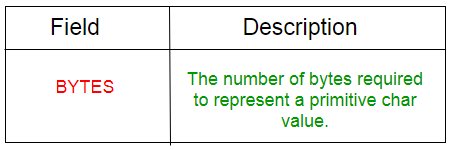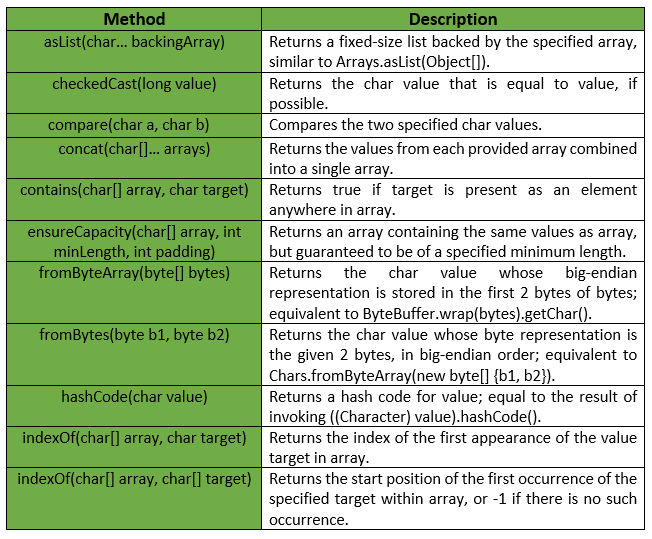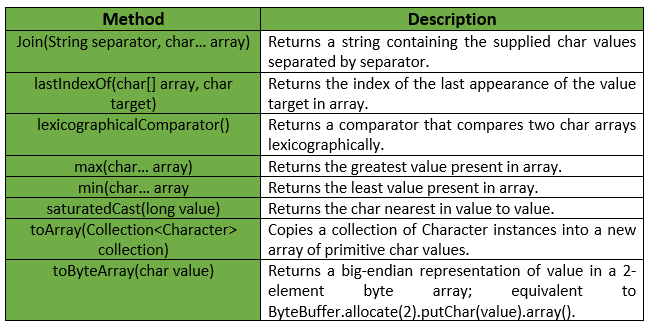# Chars Class | Guava | Java

Chars is a utility class for primitive type char. It provides Static utility methods pertaining to char primitives, that are not already found in either Character or Arrays. All the operations in this class treat char values strictly numerically, i.e, they are neither Unicode-aware nor locale-dependent.

Declaration :

```@GwtCompatible(emulated=true)
public final class Chars
extends Object
```

Below table shows the Field summary for Guava Chars Class :Some of the methods provided by Guava Chars Class are :Exceptions :

• checkedCast : IllegalArgumentException if value is greater than Character.MAX_VALUE or less than Character.MIN_VALUE
• min : IllegalArgumentException if array is empty.
• max : IllegalArgumentException if array is empty.
• fromByteArray : IllegalArgumentException if bytes has fewer than 2 elements.
• ensureCapacity : IllegalArgumentException if minLength or padding is negative.
• toArray : NullPointerException if collection or any of its elements is null.

Below table shows some other methods provided by Guava Chars Class :Below given are some examples showing the implementation of methods of Guava Chars Class :
Example 1 :

 `// Java code to show implementation ` `// of Guava Chars.asList() method ` ` `  `import` `com.google.common.primitives.Chars; ` `import` `java.util.*; ` ` `  `class` `GFG { ` `    ``// Driver method ` `    ``public` `static` `void` `main(String[] args) ` `    ``{ ` `        ``char` `arr[] = { ``'g'``, ``'e'``, ``'e'``, ``'k'``, ``'s'` `}; ` ` `  `        ``// Using Chars.asList() method which ` `        ``// converts array of primitives ` `        ``// to array of objects ` `        ``List myList = Chars.asList(arr); ` ` `  `        ``// Displaying the elements ` `        ``System.out.println(myList); ` `    ``} ` `} `

Output :

```[g, e, e, k, s]
```

Example 2 :

 `// Java code to show implementation ` `// of Guava Chars.toArray() method ` ` `  `import` `com.google.common.primitives.Chars; ` `import` `java.util.*; ` ` `  `class` `GFG { ` `    ``// Driver method ` `    ``public` `static` `void` `main(String[] args) ` `    ``{ ` `        ``List myList = Arrays.asList(``'g'``, ``'e'``, ``'e'``, ``'k'``, ``'s'``); ` ` `  `        ``// Using Chars.toArray() method which ` `        ``// converts a List of Chars to an ` `        ``// array of char ` `        ``char``[] arr = Chars.toArray(myList); ` ` `  `        ``// Displaying the elements ` `        ``System.out.println(Arrays.toString(arr)); ` `    ``} ` `} `

Output :

```[g, e, e, k, s]
```

Example 3 :

 `// Java code to show implementation ` `// of Guava Chars.concat() method ` ` `  `import` `com.google.common.primitives.Chars; ` `import` `java.util.*; ` ` `  `class` `GFG { ` `    ``// Driver method ` `    ``public` `static` `void` `main(String[] args) ` `    ``{ ` `        ``char``[] arr1 = { ``'g'``, ``'e'``, ``'e'` `}; ` `        ``char``[] arr2 = { ``'k'``, ``'s'` `}; ` ` `  `        ``// Using Chars.concat() method which ` `        ``// combines arrays from specified ` `        ``// arrays into a single array ` `        ``char``[] arr = Chars.concat(arr1, arr2); ` ` `  `        ``// Displaying the elements ` `        ``System.out.println(Arrays.toString(arr)); ` `    ``} ` `} `

Output :

```[g, e, e, k, s]
```

Example 4 :

 `// Java code to show implementation ` `// of Guava Chars.contains() method ` ` `  `import` `com.google.common.primitives.Chars; ` ` `  `class` `GFG { ` `    ``// Driver method ` `    ``public` `static` `void` `main(String[] args) ` `    ``{ ` `        ``char``[] arr = { ``'g'``, ``'e'``, ``'e'``, ``'k'``, ``'s'` `}; ` ` `  `        ``// Using Chars.contains() method which ` `        ``// checks if element is present in array ` `        ``// or not ` `        ``System.out.println(Chars.contains(arr, ``'g'``)); ` `        ``System.out.println(Chars.contains(arr, ``'m'``)); ` `    ``} ` `} `

output :

```true
false
```

Example 5 :

 `// Java code to show implementation ` `// of Guava Chars.min() method ` ` `  `import` `com.google.common.primitives.Chars; ` ` `  `class` `GFG { ` `    ``// Driver method ` `    ``public` `static` `void` `main(String[] args) ` `    ``{ ` `        ``char``[] arr = { ``'g'``, ``'e'``, ``'e'``, ``'k'``, ``'s'` `}; ` ` `  `        ``// Using Chars.min() method ` `        ``System.out.println(Chars.min(arr)); ` `    ``} ` `} `

Output :

```e
```

Example 6 :

 `// Java code to show implementation ` `// of Guava Chars.max() method ` ` `  `import` `com.google.common.primitives.Chars; ` ` `  `class` `GFG { ` `    ``// Driver method ` `    ``public` `static` `void` `main(String[] args) ` `    ``{ ` `        ``char``[] arr = { ``'g'``, ``'e'``, ``'e'``, ``'k'``, ``'s'` `}; ` ` `  `        ``// Using Chars.max() method ` `        ``System.out.println(Chars.max(arr)); ` `    ``} ` `} `

Output :

```s
```

Attention reader! Don’t stop learning now. Get hold of all the important Java and Collections concepts with the Fundamentals of Java and Java Collections Course at a student-friendly price and become industry ready.

My Personal Notes arrow_drop_upIn love with a semicolon because sometimes i miss it so badly)

If you like GeeksforGeeks and would like to contribute, you can also write an article using contribute.geeksforgeeks.org or mail your article to contribute@geeksforgeeks.org. See your article appearing on the GeeksforGeeks main page and help other Geeks.

Please Improve this article if you find anything incorrect by clicking on the "Improve Article" button below.

Article Tags :
Practice Tags :

Be the First to upvote.

Please write to us at contribute@geeksforgeeks.org to report any issue with the above content.